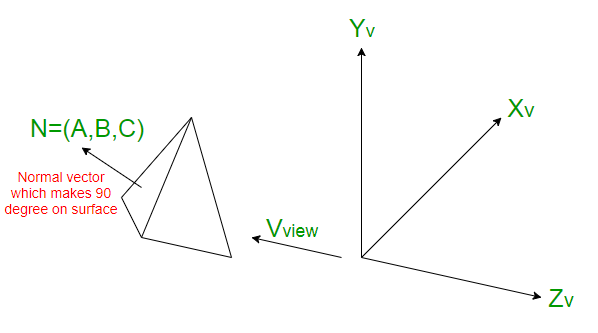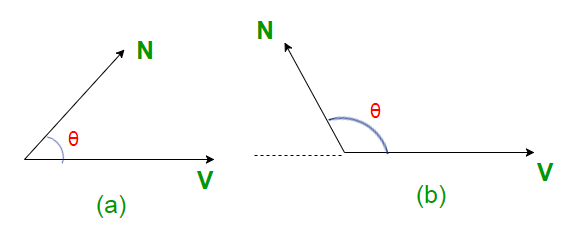# Back-Face Detection Method

When we project 3-D objects on a 2-D screen, we need to detect the faces that are hidden on 2D.
Back-Face detection, also known as Plane Equation method, is an object space method in which objects and parts of objects are compared to find out the visible surfaces. Let us consider a triangular surface that whose visibility needs to decided. The idea is to check if the triangle will be facing away from the viewer or not. If it does so, discard it for the current frame and move onto the next one. Each surface has a normal vector. If this normal vector is pointing in the direction of the center of projection, then it is a front face and can be seen by the viewer. If this normal vector is pointing away from the center of projection, then it is a back face and can not be seen by the viewer.

Algorithm for left-handed system :

```1) Compute N for every face of object.
2) If (C.(Z component) > 0)
then a back face and don't draw
else
front face and draw
```

The Back-face detection method is very simple. For the left-handed system, if the Z component of the normal vector is positive, then it is a back face. If the Z component of the vector is negative, then it is a front face.

Algorithm for right-handed system :

```1) Compute N for every face of object.
2) If (C.(Z component) < 0)
then a back face and don't draw
else
front face and draw
```

Thus, for the right-handed system, if the Z component of the normal vector is negative, then it is a back face. If the Z component of the vector is positive, then it is a front face.

Back-face detection can identify all the hidden surfaces in a scene that contain non-overlapping convex polyhedra.Recalling the polygon surface equation :

```Ax + By + Cz + D < 0
```

While determining whether a surface is back-face or front face, also consider the viewing direction. The normal of the surface is given by :

```N = (A, B, C)
```

A polygon is a back face if Vview.N > 0. But it should be kept in mind that after application of the viewing transformation, viewer is looking down the negative Z-axis. Therefore, a polygon is back face if :

```(0, 0, -1).N > 0
or if C < 0
```

Viewer will also be unable to see surface with C = 0, therefore, identifying a polygon surface as a back face if : C <= 0.Considering (a),

```V.N = |V||N|Cos(angle)
if 0 <= angle  0 and V.N > 0
Hence, Back-face.
```

Considering (b),

```V.N = |V||N|Cos(angle)
if 90 < angle <= 180, then
cos(angle) < 0 and V.N < 0
Hence, Front-face.
```

Limitations :
1) This method works fine for convex polyhedra, but not necessarily for concave polyhedra.
2) This method can only be used on solid objects modeled as a polygon mesh.

Attention reader! Don’t stop learning now. Get hold of all the important DSA concepts with the DSA Self Paced Course at a student-friendly price and become industry ready.

My Personal Notes arrow_drop_upIn love with a semicolon because sometimes i miss it so badly)

If you like GeeksforGeeks and would like to contribute, you can also write an article using contribute.geeksforgeeks.org or mail your article to contribute@geeksforgeeks.org. See your article appearing on the GeeksforGeeks main page and help other Geeks.

Please Improve this article if you find anything incorrect by clicking on the "Improve Article" button below.